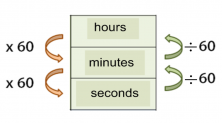Smartick is a fun way to learn math!Apr21

# Learn How to Measure Time and the Units Associated

A new measurements post! In this entry, we are going to talk about time measurement. How can we measure time? What units are used?

The tool used to measure time is the clock. The unit that we use as a reference is a day. Regarding a day, there are units of time that are smaller than a day, and there are other units of time that are greater than a day.

### Units Smaller Than The Day:

• A day has 24 hours.
• One hour has 60 minutes.
• A minute has 60 seconds.

### Units Larger Than The Day:

• 7 days make up one week.
• 15 days make up a fortnight.
• Between 28 and 31 days make up one month.
• 3 months make up a quarter.
• 6 months make up a semester.
• 12 months form one year.
• 2 years form a biennium.
• 10 years form a decade.
• 100 years form a century.
• 1000 years make up a millennium.

There are many more units used to measure time, but these are the most common.

How can we change from one unit of time to another?
In the next picture, you can see that to go from hours to minutes or to change from minutes to seconds, you must multiply by 60. On the other hand, to change from seconds to minutes or from minutes to hours, one must divide by 60.

###Time Measurement Examples:

How many minutes are 1000 seconds? To move from seconds to minutes, one must divide by 60.

1000/ 60 = 16 and 40 remain.

This means that 1000 seconds is equal to 16 minutes and 40 seconds.

How many minutes are in three hours? To go from hours to minutes we will have to multiply by 60.

3 x 60 = 180

3 hours is equal to 180 minutes

How many hours are 250,000 seconds? To change from seconds to hours one must divide by 60 twice.

250000÷60 = 4166 and 40 is remaining.

This means we have 4166 minutes and 40 seconds. Now we return to divide 60 minutes.

4166÷60 = 69 and the remainder is 26.

In the end, we find that 250,000 seconds is the same as 69 hours, 26 minutes and 40 seconds.

Register today and try Smartick for a free 15-day trial to learn more elementary math!

Fun is our brain’s favorite way of learning
Diane Ackerman
Smartick is a fun way to learn math
• 15 fun minutes a day
• Millions of students since 2009• Aliyu UbaliyoJul 23 2021, 9:39 AM

I really enjoyed this site cos it helped me too much to my study.